### Thermophysical Property Database

This database contains data on density, surface tension and viscosity coefficient of metals, alloys and ceramics obtained by floating experiments using an electrostatic levitation furnace.

The electrostatic floating method utilizes the Coulomb force acting between the charged sample and the electrode placed around it.Since it does not require a container to hold the molten liquid, mixing of impurities is prevented and accurate measurement is enabled.

This database was constructed in a JAXA-NIMS collaboration framework on materials property data utilization for academic and industrial applications (started from August 1st, 2017).Electrostatic levitation furnace

### Number of open data

Number of experiments Number of characteristic data Density measurement experiment 68 Drop vibration experiment 62 Density 68 Surface tension 55 Viscosity coefficient 60 Pure metal 72 Alloy 40 Ceramics 7

### Experiment overview

 ■ Density acquisition experiment Start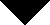① Standard sample calibration experiment② Still image segmentation 1③ Image analysis 1④ density measurement experiment⑤ sample heating / quenching⑥ Correction of melting point⑦ Still image segmentation 2⑧ Image analysis 2⑨ Volume calculation⑩ Mass measurement⑪ Density calculation⑫ Equation of temperature compensation formula⑬ Equation of density-temperature relational expressionEnd ① Standard samples (such as stainless steel) are floated and calibrated using an electrostatic levitation furnace. ② Cut out still images for image analysis from the movie data recorded in ①. ③ Analyze the image file cut out in ② and calculate the number of pixels Pixel / mm (H), Pixel / mm (V) for camera calibration.For the analysis of the image, fitting of the contour is performed by using the harmonic function represented by the following Lucian dollar polynomial Pn(cos θ).④ Suspend the sample. ⑤ Heat with reference to the melting point of the sample. ⑥ Correct the temperature measured in ⑤ with the melting point temperature during rapid cooling. ⑦ Cut out a still image for image analysis from the movie data recorded in ⑤Synchronize the cutout time with the temperature data. ⑧ Analysis of the image file cut out in ⑦ and fitting of contour The model formula is the same as ③ image analysis. The data output in the image analysis are fitting coefficients B0 to B5, sample center position x, y , And the value Rss of the error function. ⑨ The volume is calculated from the calculated R(θ). ⑩ The sample is collected and the mass is measured. ⑪ Density is calculated from the calculated volume and mass. ⑫ Make a regression equation based on the temperature data corrected by the temperature melting point during quenching. ⑬ An approximate expression of the density-temperature relation is made using the calculated density and temperature data. ■Droplet vibration experiment Start① Surface tension / viscosity coefficient Measurement experiment② Droplet vibration experiment③ Still image segmentation 3④ Image analysis 3⑤ sample radius / flatness ratio calculated⑥ Simple analysis⑦ Nonlinear Fitting⑧ Identification of sample rotation speed⑨ Correction of rotation⑩ Correction of charge / gravity⑪ Surface tension calculation⑫ Calculation of viscosity coefficientEnd ① Suspend the sample. ② Apply voltage to excite droplet vibration The excited vibration is the second order vibration mode as shown below, then cut off and attenuate the voltage.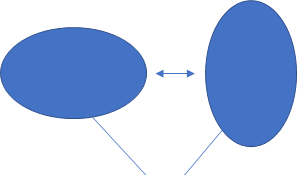sample ③ Choose beautiful waveform data from the sample movie data when droplet vibration of ② and extract the still image. ④ Analyze the sample image The procedure is the same as ③ in Density experiment. Calculate shape approximation coefficient, pixel number dh, dv. ⑤ Calculate sample radius and oblateness from the result of ⑤. ⑥ Calculate the resonance frequency ω and the damping coefficient τ using the vibration data and the model equation by simple analysis When the shape of the sample undergoes minute deformation of the axis target as shown in the above figure, the time change r(t) Is described as follows.r 0 is the sample diameter at the time of spherical formation and is calculated by ⑤.P n (cosθ) is an nth order Lucian polynomial, θ is the angle between the z axis direction and the radial direction, and r n is the amplitude according to the n th order vibration mode.At this time, the resonance frequency ω n and the resonance coefficient τ n are expressed as follows using the expression of Rayleigh * .σ is the surface tension of the droplet, p is the density, and η is the viscosity coefficient.Here, substituting n = 2 in this experiment gives the resonance frequency ω 2 and the damping coefficient τ 2 as follows.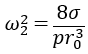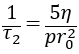⑦ Fitting is performed to increase the frequency when the amplitude of the droplet is large by FFT analysis using the result obtained by the simple analysis of ⑥.In the above equation, A represents the amplitude and Φ represents the phase shift, and the resonance frequency ω 2 is as follows.⑧ In order to correct the influence of the rotation of the sample, frequency shift ⊿ω (%) and damping coefficient shift ⊿τ (%) is calculated from the flattening ratio. ⑨ Calculate 2.23 * τ as the time when the attenuation is the first 1/10, and calculate the final ω and τ using ⊿ω and ⊿τ calculated in ⑧. ⑩ Correct errors due to surface charge and gravity effects.Charge correction by Rayleigh * model is given by the following equation.In addition, the correction of gravity is as follows.Q s is a charge, and ε 0 is a vacuum dielectric constant.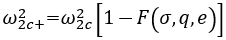⑪ Calculate the viscosity coefficient from sample radius, density, τ. ⑫ Surface tension is calculated from sample radius, density, ω.

## NEWS

• 2019.09.02 News MatNavi Thermophys